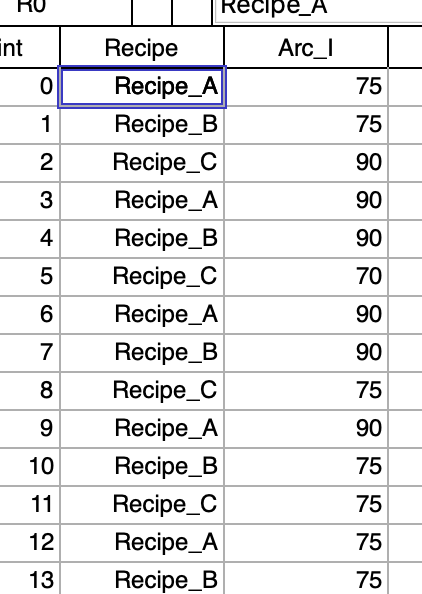# Wave Reference in Function CallHi,

I have put together a little function to help create box plots. And it seems to do the job nicely.

The idea is that I have a couple of waves, one containing the data and a second containing a text based description.and the idea is to group by the text label and use those labels in a box plot.  All good here and now I want to take it to the next level and be able to use a numeric wave as the grouping and label.  The example would be if I had an experimental condition in a wave such as a temperature setting.  I would like to use the numeric value as an ordinal/nominal value instead of a continuous one.  The code to remap from numeric to text is straightforward.

Now the question: I would like to have a single function to call and have the use of a text wave or the needed remapping within the function.  In my code the two waves passed by reference include the numeric wave of the data and the second is the labelwave which is now set to text in the declaration.  Is it possible to pass a wave reference with declaring the type immediately and then determining the type with in the body of function and proceed accordingly?  So I an wondering if I can set the labelwave to be either text or numeric in the pass reference and then use wavetype to decide how it is handled?

Hope that is a clear question.

Andy

Function NewBox(Inwave,LabelWave)
wave Inwave
wave/T labelwave

Findduplicates/FREE /RT=textlabels Labelwave

variable index,maxindex,points,maxpoints
maxindex= numpnts(textLabels)

Make/O /n=(numpnts(Inwave),maxindex) \$(nameOfWave(inWave)+"_box")
Wave results = \$(nameOfWave(inWave)+"_box")
results=NaN
For(index=0;index<maxindex;index+=1)
SetDimLabel 1,index,\$cleanupName(textlabels[index],0),results
extract/FREE inWave, tempwave, !cmpstr(labelwave,textlabels[index])
points = numpnts(tempwave)-1
results[0,points][index]=tempwave[p]
maxpoints = max(maxpoints,points)
endfor
redimension /N=(maxpoints,-1) results
Display;AppendBoxPlot/CATL results
end

How about having a text wave with numbers in this case? This way you could pass this into your function without jumping through hoops. A clumsy way to achieve what you want could be to pass the path to your label wave as a string to the function and then do this:

Variable Type = WaveType(\$pathToWave)
if (Type == 0)  // text wave
...
else
...
endif

You could then convert the wave to text internally in your function. However, it is not possible to just switch between Wave and Wave/T declarations in the if statement, so it will be fiddly to handle inside the code.

Yes this can be done. The type of the wave is not fixed at compile time. It is a mere hint (yes this is simplified).

The following example illustrates that:

Function PrintMe(WAVE/Z wv)
//  assumes wv is not emtpy

variable var
string str

switch(WaveType(wv, 1))
case 0:
print "null wave"
break
case 1:
var = wv
printf "Numeric wave: %g\r", var
break
case 2:
WAVE/T wvText = wv
str = wvText
printf "Text wave: %s\r", str
break
default:
// and so on
break
endswitch
End

And feeding in some data gives

make/o data = p
make/T/o dataText = num2str(p^2)
•printme(\$"")
null wave
•printme(data)
Numeric wave: 0
•printme(dataText)
Text wave: 0

One noteworthy thing is that we have to declare the wave wvText with /T so that we can read from it into a string. The same approach would work if wave is a wave reference wave or datafolder reference wave.